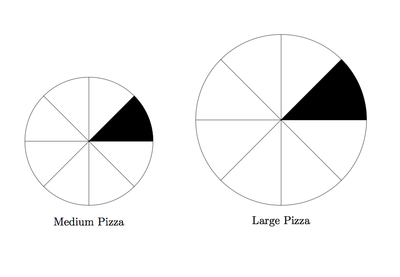# Comparing two different pizzas

Alignments to Content Standards: 4.NF.B

Jessica and some friends have ordered two pizzas. One is a medium sized pizza while the other is a large.Jessica eats two slices of the medium sized pizza. Has Jessica eaten $\frac{2}{16}$ of the two pizzas? Explain your reasoning, and draw a picture to illustrate your explanation.

## IM Commentary

The focus of this task is on understanding that fractions, in an explicit context, are fractions of a specific whole. In this this problem there are three different wholes: the medium pizza, the large pizza, and the two pizzas taken together. Because the medium and large pizza are not the same size and Jessica has taken two slices of the medium size pizza it is not the case that she has eaten $\frac{2}{16} = \frac{1}{8}$ of the two pizzas. The two pizzas together can be naturally divided into $8$ equal portions but this would mean taking one piece of the medium and one piece of the large pizza.

This task is best suited for instruction. Students can practice explaining their reasoning to each other in pairs or as part of a whole group discussion.

## Solution

Below is a picture of the pizza which Jessica ate:The fraction $\frac{2}{16}$ represents two out of $16$ equal pieces making up a whole. For this problem, the whole is the medium and the large pizza taken together. While it is true that Jessica has eaten $2$ of the $16$ pieces of pizza, the $16$ pieces are not all the same size. In particular, each piece does not represent $\frac{1}{16}$; the smaller pieces are less than $\frac{1}{16}$ and the larger pieces are greater than $\frac{1}{16}$.

Jessica has had two of the smaller pieces and so in fact has had less than $\frac{2}{16}$ of the two pizzas. To see this more clearly, note that $\frac{2}{16} = \frac{1}{8}$. The two pizzas can be evenly divided into $8$ equal portions if each portion consists in one slice of the medium pizza and one slice of the large pizza:Looking at the two pictures we can see that Jessica has had less than $\frac{2}{16} =\frac{1}{8}$ of the two pizzas: one piece of the medium pizza was correct but for her second piece she would have needed to eat a piece of the large instead of a (smaller) piece of the medium pizza.